# box function and types of boxes with bty

## The `box` function

The R `box` function allows adding a box around plots. This is particularly useful when you add a new axis with the `axis` function and want to draw the box again. You can also customize the color, the line type, the line width and even the type of the box.

Example 1. Adding a blue box to the plot. Note that we set `axes = FALSE` to avoid displaying the default box and axes.

``````# Plot without axes
curve(cos, -10, 10, axes = FALSE)

# Blue dashed box
box(col = 4, lty = 2)``````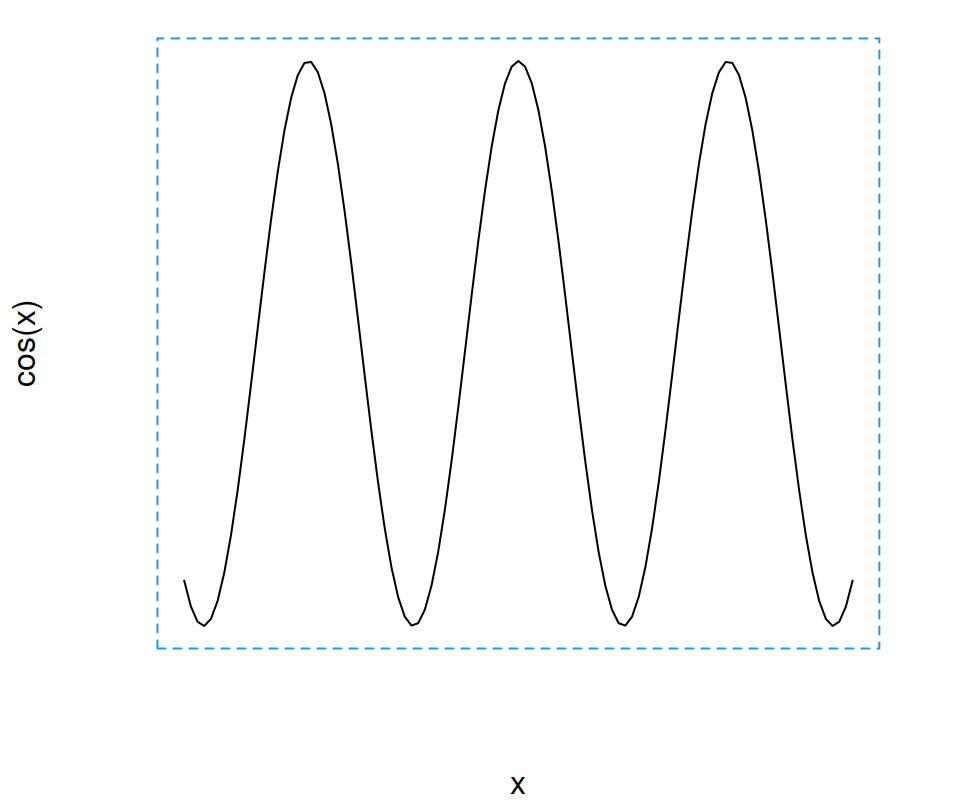Example 2. Adding a box after modifying the axes with the `axis` function.

``````# Plot without axes
curve(cos, -10, 10, axes = FALSE)

# Custom axis
axis(1, at = c(-2 * pi, pi, -pi, 0, 2 * pi),
labels = expression(-2 * pi, -pi, 0,
pi, 2 * pi))

axis(2, lwd = 2, lty = 2)

# Box with wide line
box(lwd = 2)``````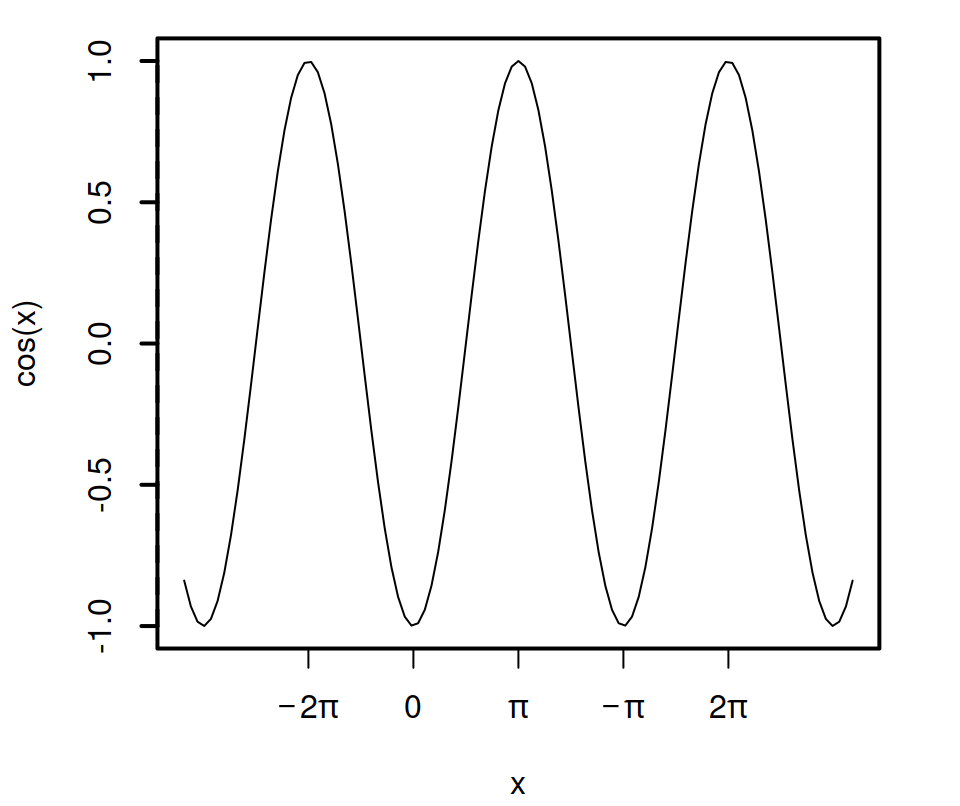Example 3. Adding a box to the whole plot.

``````# Plot without axes
curve(cos, -10, 10)

# Border box
box("figure", col = 4, lwd = 4)``````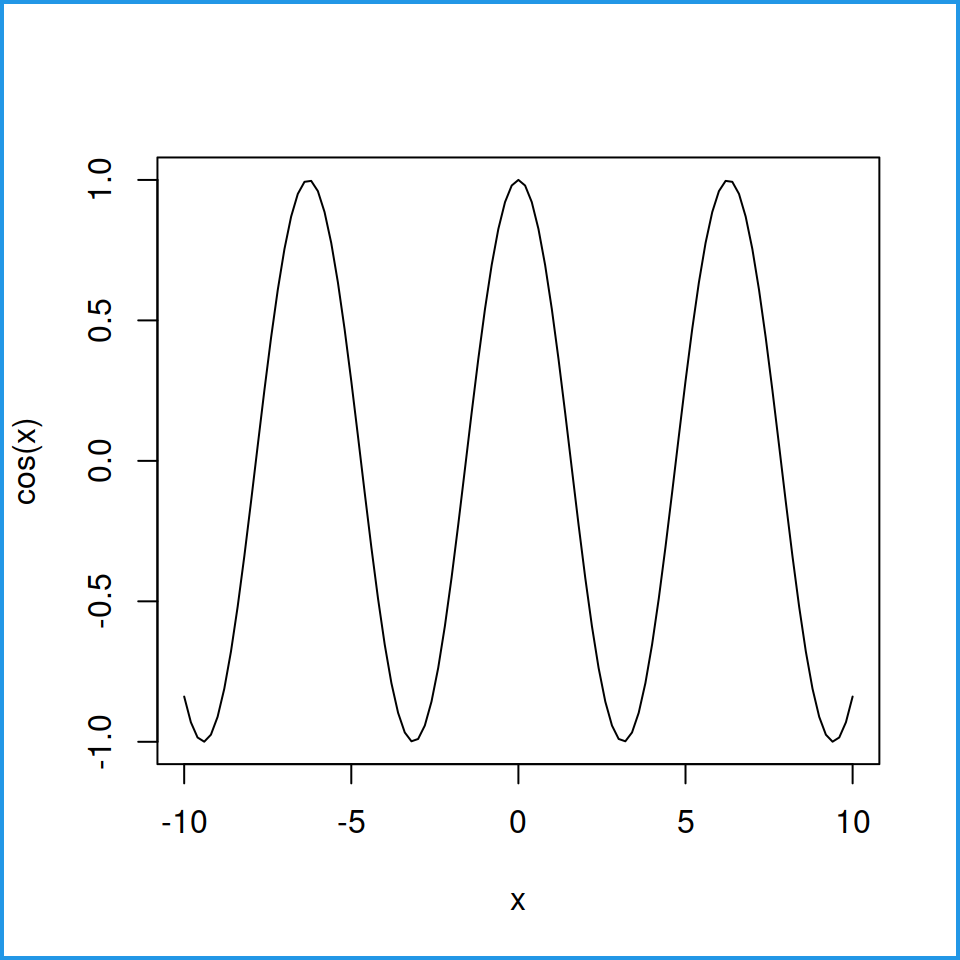## Box type with `bty` argument

By default, base R plots have a box around them. This box can be customized with the `bty` argument of the corresponding function. If the plotting function does not support this argument (like `boxplot`) you can set it inside `par` function.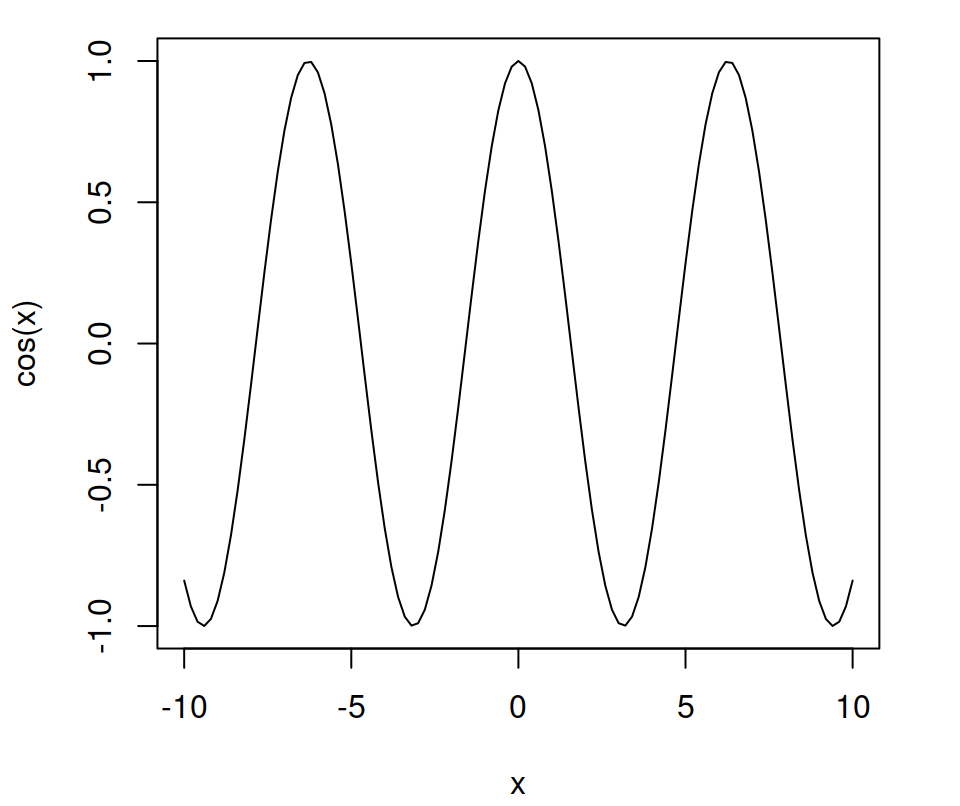Full box: `bty = "o"` (Default)

``````curve(cos, -10, 10,
bty = "o")``````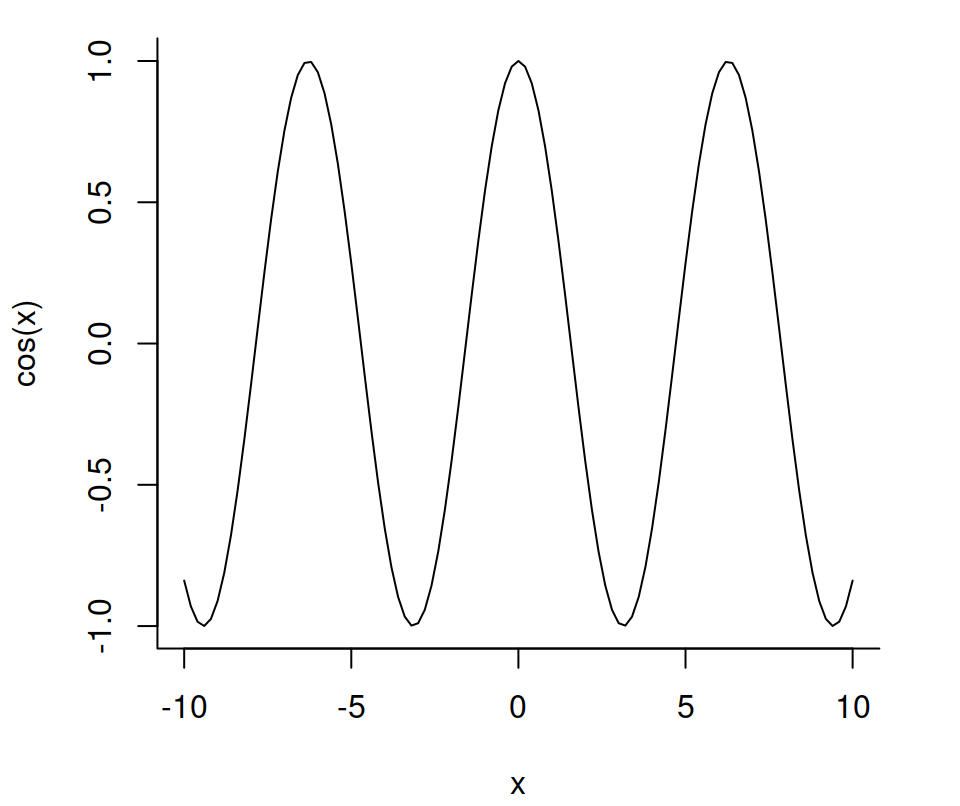Left and bottom: `bty = "L"`

``````curve(cos, -10, 10,
bty = "L")``````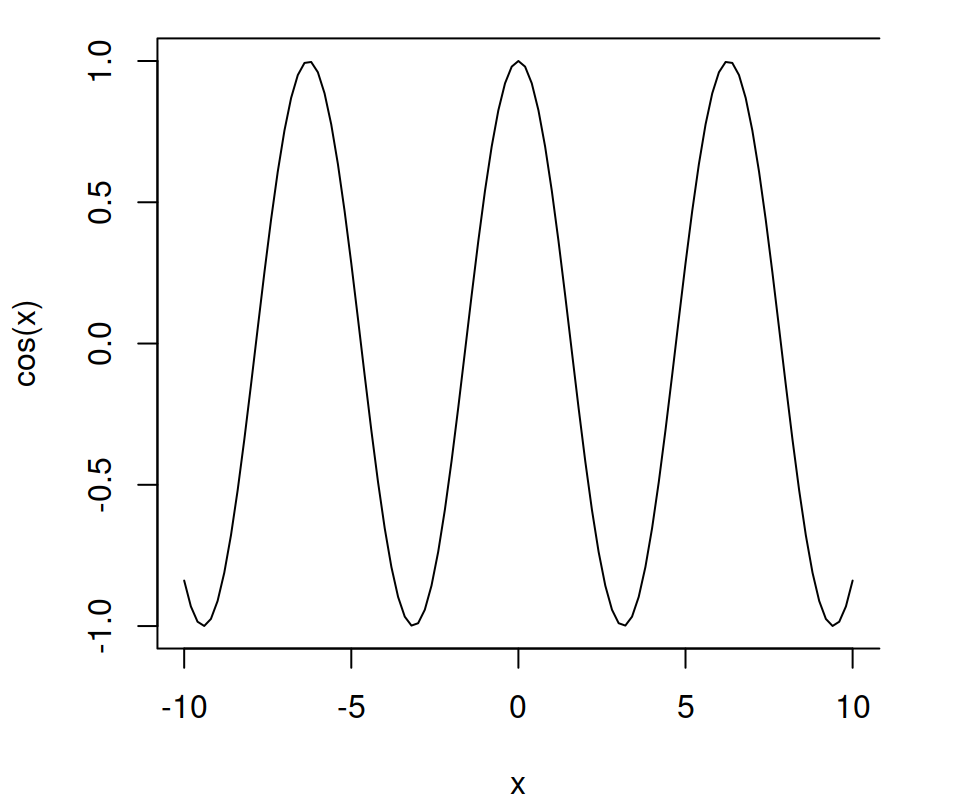Top, left and bottom: `bty = "C"`

``````curve(cos, -10, 10,
bty = "C")``````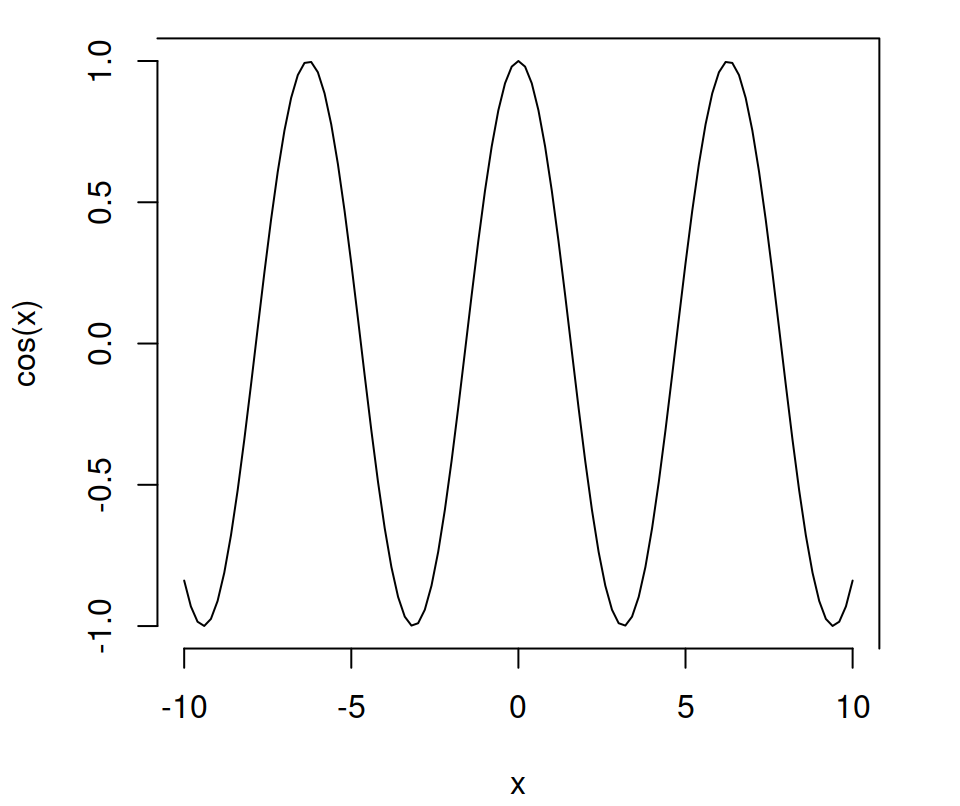Top and right: `bty = "7"`

``````curve(cos, -10, 10,
bty = "7")``````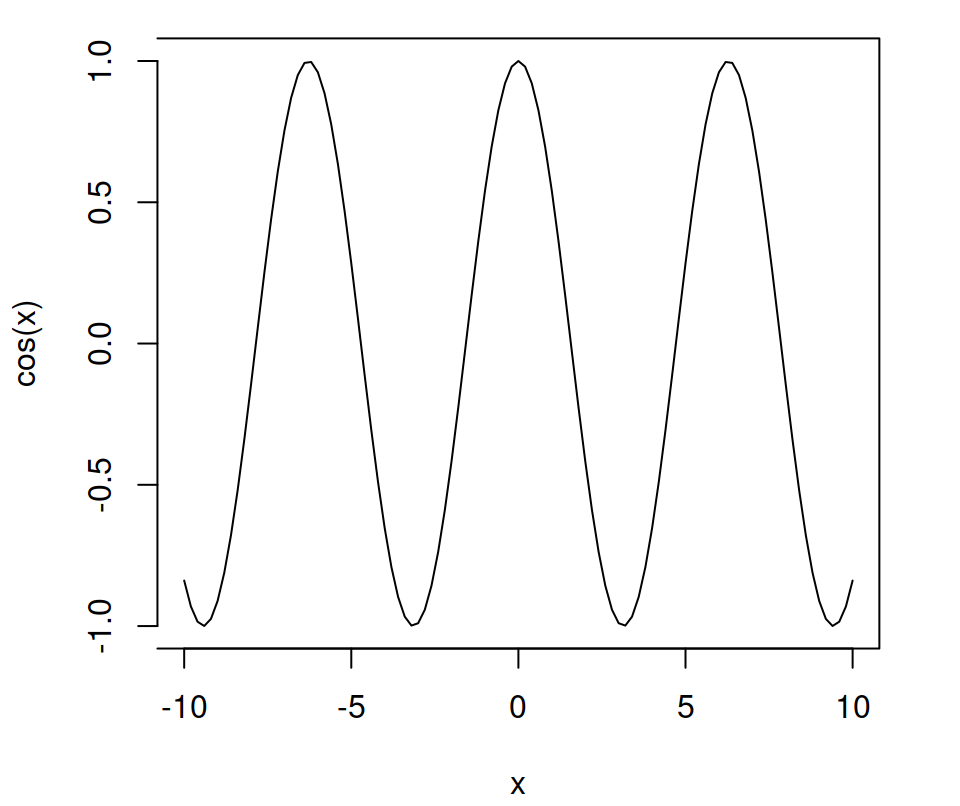Top, right and bottom: `bty = "]"`

``````curve(cos, -10, 10,
bty = "]")``````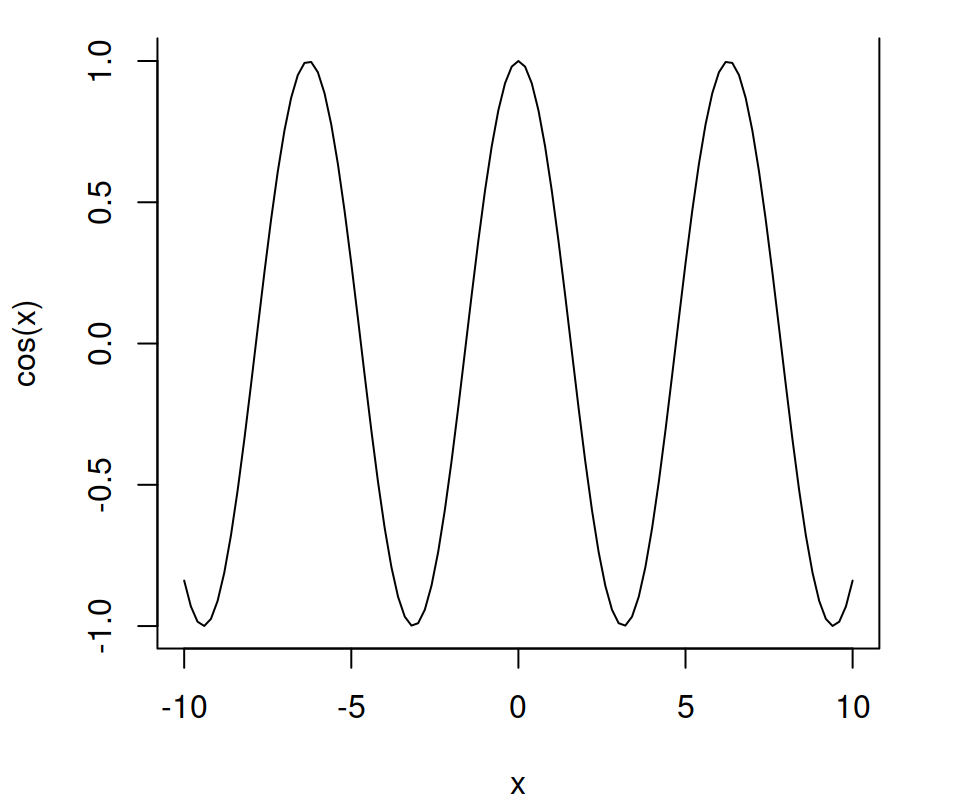Left, bottom and right: `bty = "U"`

``````curve(cos, -10, 10,
bty = "U")``````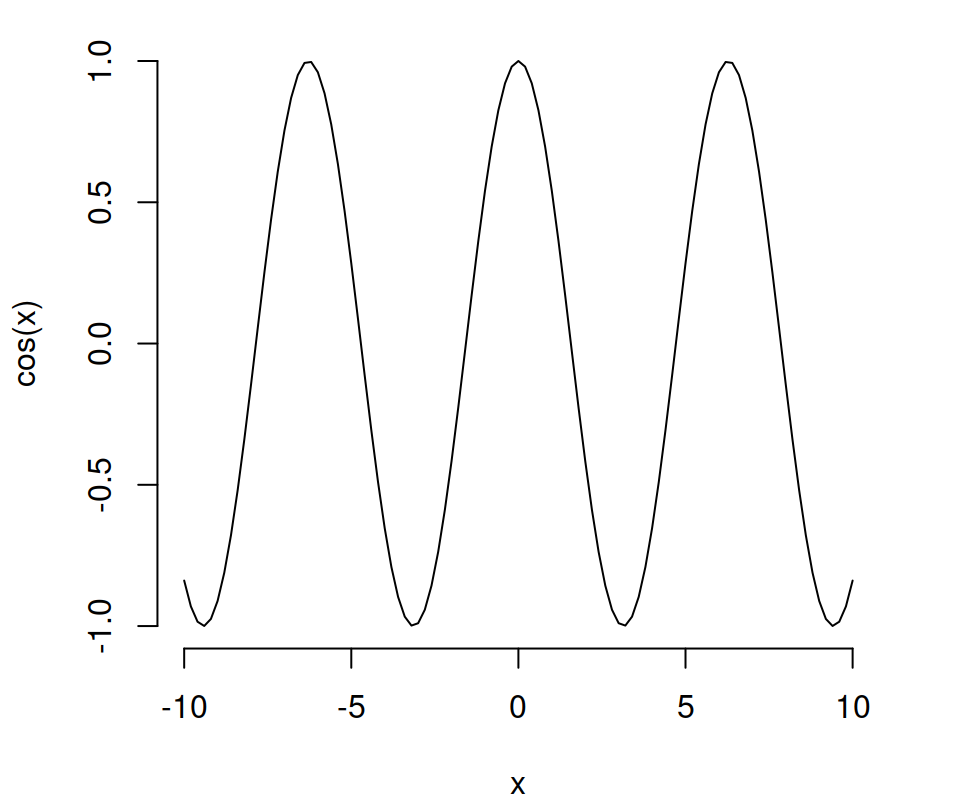No box: `bty = "n"`

``````curve(cos, -10, 10,
bty = "n")``````

The `bty` argument can also be used within the `box` function, which allows customizing the color, the type and the width of the box.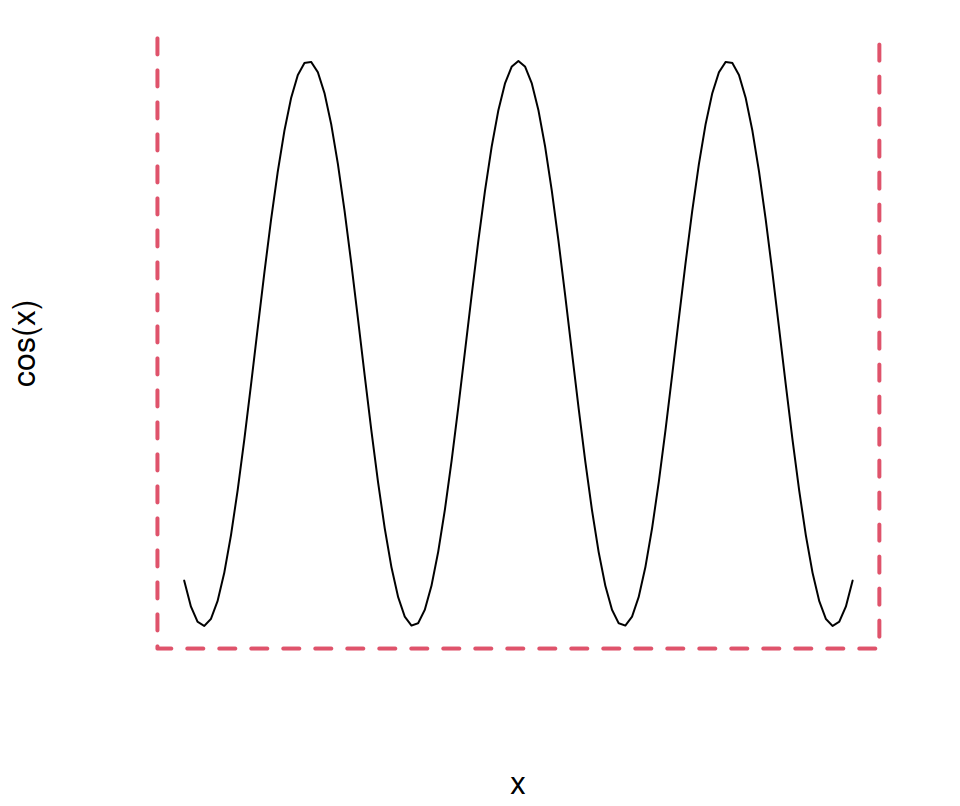``````curve(cos, -10, 10,
axes = FALSE)

box(col = 2, bty = "U", lty = 2, lwd = 2)``````

As we pointed out before, with some functions you will need to specify the argument inside the `par` function. As an example, if you want to draw a box plot without a box you can type: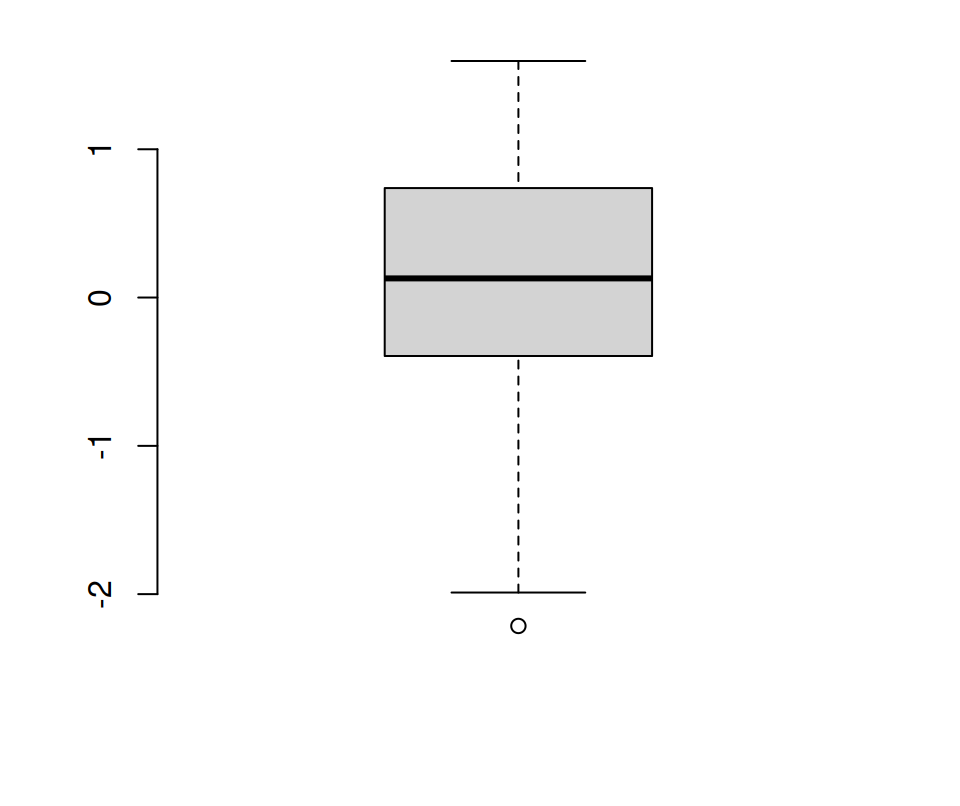``````par(bty = "n")

# Data
set.seed(1)
x <- rnorm(50)

# Plot
boxplot(x)``````

Note that `"o"`, `"7"`, `"]"`, `"L"` and `"U"` represent the sides of the box that are actually drawn. This is a good way to remember them. You can also set the letters in lower case instead of in upper case.